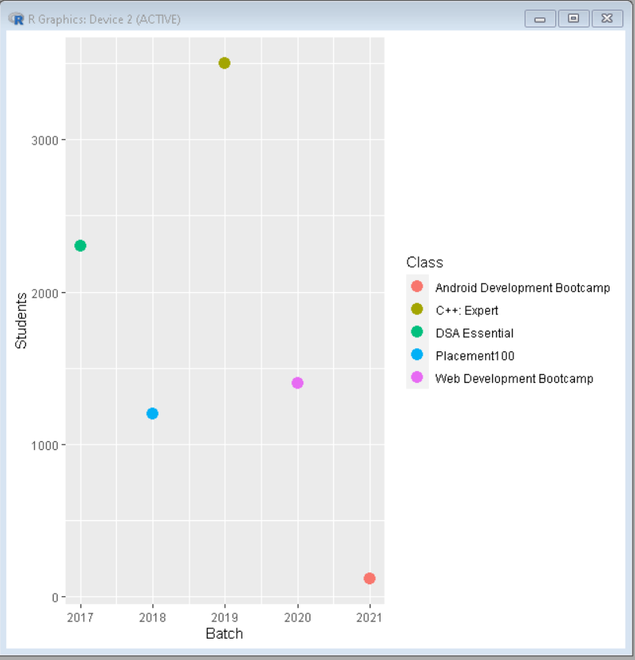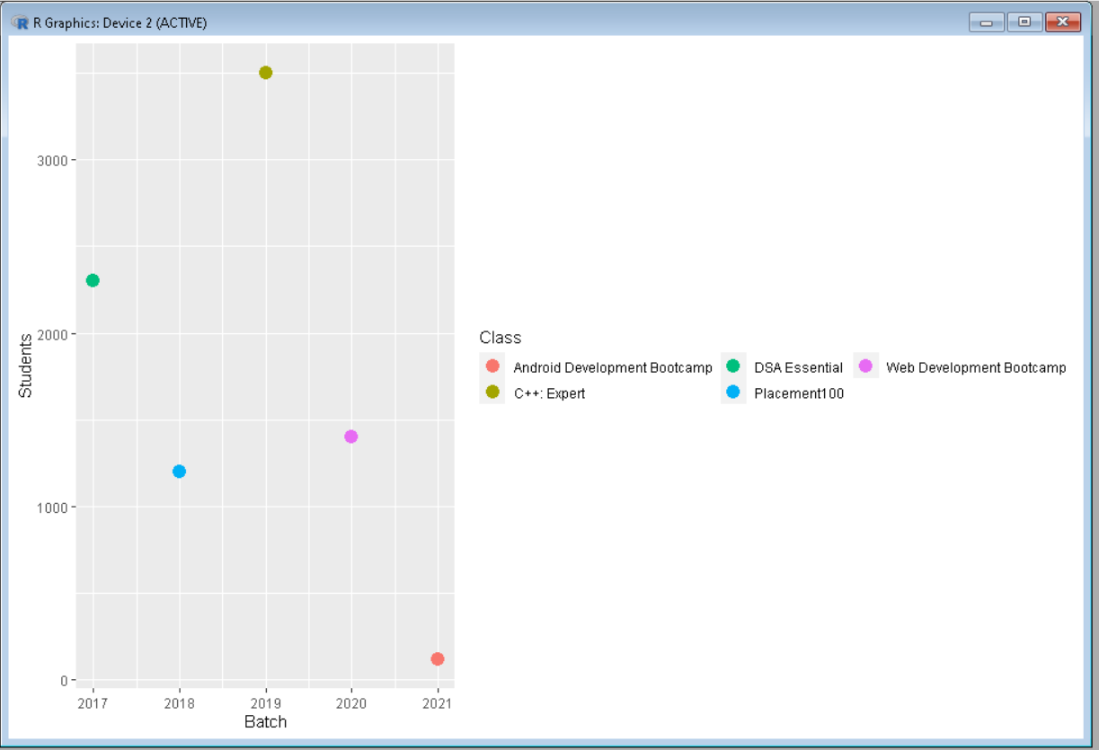Related Articles

# How To Fold Legend into Two Rows in ggplot2 in R

• Last Updated : 14 Sep, 2021

In this article, we are going to see how to draw a ggplot2 legend with two Rows in R Programming Language.

If we want to draw ggplot2 Legend with two rows, we have to add guides and guide_legend functions to the theme() function. Inside guides() function, we take parameter named color, which has call to guide_legend() guide function as value.

Inside the guide_legend() function, we take an argument called nrow, which has the desired number of rows of legend as value.

Syntax : guide_legend(nrow)

Parameter :

nrow : The Desired Number of rows of legend.

Return : Legend Guides for various scales

Dataframe in use:

```  Batch Students                        Class
1  2017     2300                DSA Essential
2  2018     1200                 Placement100
3  2019     3500                  C++: Expert
4  2020     1400     Web Development Bootcamp
5  2021      120 Android Development Bootcamp```

To Create an R plot, we use ggplot() function, and to make it a scatter plot we add geom_point() function, assign this whole plot to gplot data object. Let us first create a regular plot so that the difference is apparent.

Example: Default legend

## R

 `library``(``"ggplot2"``)``   ` `# Create a DataFrame``data <- ``data.frame``(Batch = ``c``(2017, 2018, 2019, 2020, 2021),``                   ``Students = ``c``(2300, 1200, 3500, 1400, 120),``                   ``Class = ``c``(``"DSA Essential"``, ``"Placement100"``,``                             ``"C++: Expert"``, ``"Web Development Bootcamp"``,``                             ``"Android DevelopmentBootcamp"``))`` ` `# Create a ggplot2 scatter plot``ggplot``(data, ``aes``(Batch, Students, color = Class)) +``geom_point``(size = 4)`

Output:Now to fold the legend, add guides() function with color as guide_legend() together with parameter nrow=2, which folds legend into two-row.

Example: Legend folded into 2 rows

## R

 `library``(``"ggplot2"``)``   ` `# Create a DataFrame``data <- ``data.frame``(Batch = ``c``(2017, 2018, 2019, 2020, 2021),``                   ``Students = ``c``(2300, 1200, 3500, 1400, 120),``                   ``Class = ``c``(``"DSA Essential"``, ``"Placement100"``, ``                             ``"C++: Expert"``, ``"Web Development Bootcamp"``, ``                             ``"Android Development Bootcamp"``))`` ` `# Create a ggplot2 scatter plot``ggplot``(data, ``aes``(Batch, Students, color = Class)) + ``geom_point``(size = 4) + ``guides``(color = ``guide_legend``(nrow = 2))`

Output:My Personal Notes arrow_drop_up